# Fourier Series

(redirected from Fourier sine series)
Also found in: Dictionary, Thesaurus.
Related to Fourier sine series: Cosine series, Fourier cosine series, Wolfram Alpha

## Fourier series

[‚fu̇r·ē‚ā ‚sir·ēz]
(mathematics)
The Fourier series of a function ƒ(x) is Also known as Fourier expansion.
McGraw-Hill Dictionary of Scientific & Technical Terms, 6E, Copyright © 2003 by The McGraw-Hill Companies, Inc.
The following article is from The Great Soviet Encyclopedia (1979). It might be outdated or ideologically biased.

## Fourier Series

a trigonometric series that provides a decomposition of a periodic function into its harmonic components. If a function f(x) is periodic with period 2T, then its Fourier series has the form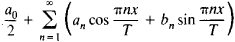and a0, an, and bn (n > 1) are its Fourier coefficients. Depending on the nature of the integrals in the formulas for the Fourier coefficients, we speak of Fourier-Riemann series, Fourier-Lebesgue series, and so on. Periodic functions with period 2π are usually considered; the general case reduces to this case by a transformation of the independent variable.

Fourier series are the simplest class of expansions with respect to an orthogonal system of functions, namely, the trigonometric system 1, cos x, sin x, cos 2x, sin 2x,. . ., cos nx, sin nx. This system of functions possesses the important properties of closure and completeness. The Fourier sums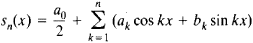minimize the integral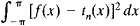where tn(x) is an arbitrary trigonometric polynomial of order ≤ n and f(x) is square integrable. Here,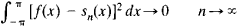so that the square integrable function f(x) can be approximated in the means by its Fourier sums with an arbitrary degree of closeness (seeAPPROXIMATION AND INTERPOLATION OF FUNCTIONS).

B. Reimann and H. Lebesgue showed that if f(x) is integrable then its Fourier coefficients an and bn tend to zero as x → ∞. Reimann also established that if f(x) has an improper Riemann integral then its Fourier coefficients need not tend to zero. If f(x) is square integrable, then the series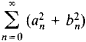converges and we have the Parseval equality: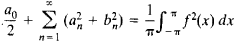One variant of this equality was discovered by the French mathematician M. Parseval in 1799; the general formula (with the integral denoting the Lebesgue integral) was proved by Lebesgue. Conversely, given a sequence of real numbers an, bn for which the series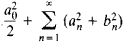converges, we can find a square integrable (in the sense of Lebesgue) function with the Fourier coefficients an and bn; this result is due to the Hungarian mathematician F. Riesz and the German mathematician E. Fischer. For Riemann integrals the Riesz-Fischer theorem is false.

There are many convergence criteria for Fourier series, that is, sufficient conditions that guarantee the convergence of the series. The following condition, for example, is due to P. Dirichlet: if f(x) has a finite number of minima and maxima on a period interval, then its Fourier series converges everywhere. A more general condition is that of C. Jordan: if f(x) is of bounded variation (seeVARIATION OF A FUNCTION), then its Fourier series converges everywhere, and the convergence is uniform on every interval contained in the interval of continuity of f(x). The Italian mathematician U. Dini proved the following criterion in 1880: if f(x) is continuous and its modulus of continuity ω(δ, f) satisfies the condition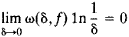then its Fourier series converges uniformly.

The comprehensive investigation of convergence conditions of Fourier series is very difficult, and final results have not yet been obtained. Riemann showed that the convergence or divergence of a Fourier series at a point x0 depends on the behavior of the corresponding function f(x) in an arbitrarily small neighborhood of x0 (the localization principle for Fourier series). If different limits f(x0 – 0) and f(x0 + 0) exist and the Fourier series converges at x0, then it converges to {f(x0 – 0) + f(x0 + 0)}/2. In particular, if the Fourier series of a continuous periodic function f(x) converges everywhere, then its sum is f(x).

The German mathematician P. du Bois-Reymond showed in 1875 that there exist continuous functions whose Fourier series diverge at infinitely many points. Furthermore, A. N. Kolmogo-rov proved in 1926 that there exist Lebesgue integrable functions whose Fourier series diverge everywhere. L. Carleson, however, established in 1966 that the Fourier series of a square integrable function converges almost everywhere. As R. Hunt showed in 1968, this result holds for functions in any space Lp(–π, π) with p < 1. These “convergence defects” gave rise to various summability methods of Fourier series. Investigation of the behavior of Fourier sums is replaced by the study of their means, which in many cases exhibit far more regular behavior. For example, L. Fejér proved in 1904 the following: if f(x) is a continuous periodic function, then for n → ∞ its Fejér sum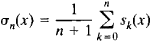converges uniformly to f(x).

### REFERENCES

Tolstov, G. P. Riady Fur’e, 2nd ed. Moscow, 1960.
Bari, N. K. Trigonometricheskie riady. Moscow, 1961.
Zygmund, A. Trigonometricheskie riady, vols. 1–2. Moscow, 1965. (Translated from English.)